# GSEB Solutions Class 8 Maths Chapter 13 Direct and Inverse Proportions Ex 13.1

Gujarat Board GSEB Textbook Solutions Class 8 Maths Chapter 13 Direct and Inverse Proportions Ex 13.1 Textbook Questions and Answers.

## Gujarat Board Textbook Solutions Class 8 Maths Chapter 13 Direct and Inverse Proportions Ex 13.1

Question 1.
Following are the car parking charges near a railway station uptoCheck if the parking charges are in direct proportion to the parking time.
Solution:
We have: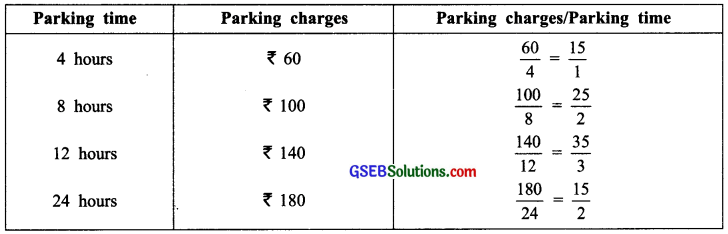Since $$\frac{15}{1}$$ ≠ $$\frac{15}{2}$$ ≠ $$\frac{35}{3}$$ ≠ $$\frac{15}{2}$$
∴ The parking charges are not in direct proportion with the parking time.Question 2.
A mixture of paint is prepared by mixing 1 part of red pigments with 8 parts of base. In the following table, find the parts of base that need to be added?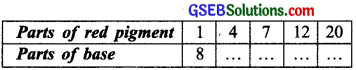Solution:
Let the red pigment be represented by x1, x2, x3, … and the base represented by y1, y2, y3 …………..
As the base increases, the required number of red pigments will also increase.
∴ The quantities vary directly.Question 3.
In question 2 above, if 1 part of a red pigment requires 75 mL of base, how much red pigment should we mix with 1800 mL of base?
Solution:
We have
$$\frac{x_{1}}{y_{1}}$$ = $$\frac{1}{8}$$ = $$\frac{x_{2}}{y_{2}}$$
Here, x1 = 1, y1 = 75 and y2 = 1800
∴ $$\frac{1}{75}$$ = $$\frac{x_{2}}{1800}$$
⇒ x2 = $$\frac{1800}{75}$$ = 24
Thus, the required red pigments = 24 parts.Question 4.
A machine in a soft drink factory fills 840 bottles in six hours. How many bottles will it fill in five hours?
Solution:For more number of hours, more number of bottles would be filled. Thus given quantities vary directly.
∴ $$\frac{840}{x}$$ = $$\frac{6}{5}$$
or 6x = 5 × 840
⇒ x = $$\frac{5×840}{6}$$
= 5 × 140 = 700
Thus, the required number of bottles = 700

Question 5.
A photograph of a bacteria enlarged 50,000 times attains a length of 5 cm as shown in the diagram. What is the actual length of the bacteria? If the photograph is enlarged 20,000 times only, what would be its enlarged length?Solution:
Let the actual length of the bacteria be x cm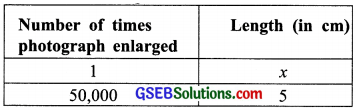The length increases with an increment in the number of times the paragraph enlarged.
∴ It is a case of direct proportion
∴ $$\frac{1}{50000}$$ = $$\frac{x}{5}$$ or x = $$\frac{5}{50000}$$
= $$\frac{1}{10000}$$ cm = 10-4 cm
Again,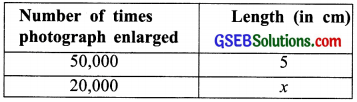We have $$\frac{5}{50000}$$ = $$\frac{x}{20000}$$
or 50000 × x = 5 × 20000
or x = $$\frac{5×20000}{50000}$$ = 2
Thus, the enlarged length = 2 cmQuestion 6.
In a model of a ship, the mast is 9 cm high, while the mast of the actual ship is 12 m high. If the length of the ship is 28m, how long is the model ship?Solution:
Let the required length of the model of the ship be x cm.
We have: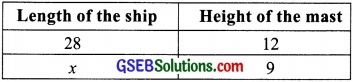Since, more the length of the ship, more would be the length of its mast.
∴ It is a case of direct variation.
∴ $$\frac{28}{x}$$ = $$\frac{12}{9}$$
or x = $$\frac{28×9}{12}$$ = 7 × 3 = 21
Thus, the required length of the model = 21 cm

Question 7.
Suppose 2 kg of sugar contains 9 × 106 crystals. How many sugar crystals are there in

1. 5 kg of sugar? and
2. 1.2 kg of sugar?

Solution:
Let the required number of sugar crystals be x in 5 kg of sugar.
We have:
1.Since, more the amount of sugar, more would be the number of sugar crystals.
∴ It is a case of direct variation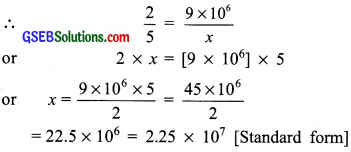Thus, the required number of sugar crystals = 2.25 × 107

2. Let the number of sugar crystals in 1.2 kg of sugar be y.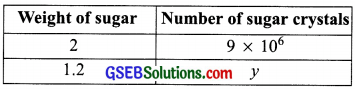∴ For a direct variation,
$$\frac{2}{1.2}$$ = $$\frac{9 \times 10^{6}}{y}$$
or 2 × y = 1.2 × 9 × 106
or y = $$\frac{12}{10}$$ × $$\frac{9}{2}$$ × 106 = 5.4 × 106
Thus, the required number of sugar crystals = 5.4 × 106Question 8.
Rashmi has a road map with a scale of 1 cm representing 18 km. She drives on a road for 72 km. What would be her distance covered in the map?
Solution:
Let the required distance covered in the map be x cm.
We have: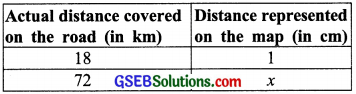Since, it is a case of direct variation,
∴ $$\frac{18}{72}$$ = $$\frac{1}{x}$$ or 18 × x = 1 × 72
or x = $$\frac{1×72}{18}$$ = 1 × 4 = 4
Thus, the required distance on the map is 4 cm.

Question 9.
A 5 m 60 cm high vertical pole casts a shadow 3 m 20 cm long. Find at the same time.

1. The length of the shadow cast by another pole 10 m 50 cm high and
2. The height of a pole which casts a shadow 5 m long.

Solution:
1. Let the required length of shadow be x cm.
We have: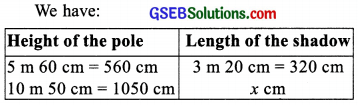Height of the pole Length of the shadow
As the height of the pole increases the length of its shadow also increases in the same ratio.
Therefore, it is a case of direct variation.
∴ $$\frac{560}{1050}$$ = $$\frac{320}{x}$$
or 560 × x = 320 × 1050
or x = $$\frac{320×1050}{560}$$
= 4 × 150 = 600 cm
Thus, the required length of the shadow = 600 cm or 6 m

2. Let thc required height of the pole be y cm.
∴ We have: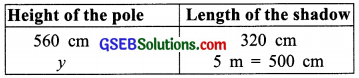Since, it is a case of direct vanation.
∴ $$\frac{560}{y}$$ = $$\frac{320}{500}$$
or 320 × y = 500 × 500
or y = $$\frac{500×560}{320}$$
= 125 × 7 = 875
Thus, the required height of the pole = 875 cm or 8 m 75 cm.Question 10.
A loaded truck travels 14 kM in 25 minutes. If the speed remains the same. how far can it travel in 5 hours?
Solution:
Since thc speed is constant,
∴ For longer distance, more time will be required
So, it is a case of direct variation.
Let the required distance to be travelled in 5 hours (= 300 minutes) be x km.
We have: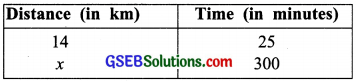∴ $$\frac{14}{x}$$ = $$\frac{25}{300}$$
or 25 × x = 14 × 300
or x = $$\frac{14×300}{25}$$ = 14 × 2 = 168
∴ The required distance = 168 km.